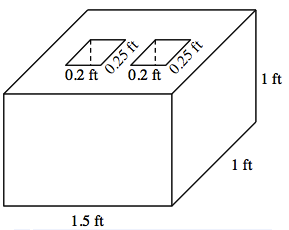### Home > CCG > Chapter 10 > Lesson 10.2.2 > Problem10-80

10-80.

A cement block is the shape of a prism with length $1.5$ ft, width $1$ ft and height $1$ ft. Centered on the top of the block and passing all the way through the block are two $0.25$ ft by $0.2$ ft rectangular holes.

1. Draw a diagram of the block.

2. What is the volume of the block?

•• Subtract the volumes of the two inner prisms from the volume of the large outer prism.